# Vehicle Suspension System camp-g, matlab and simulink model

 Vehicle Suspension System CAMP-G, MATLAB and SIMULINK Model Sacramento State - MSME Program ME270 Fall 2007 By: Justin Otero Advisor: Prof. Granda Introduction: The simulation of a vehicles suspension system represents an important part of how the driver experiences ride quality. Without a suspension system, a vehicle acts in a stiff and uncomfortable way. The characteristics of a vehicles performance are dependent on the properties of the suspension. A model of this system would enable a manufacturer to test how certain changes to the properties change the behavior of the vehicle. This way they are able to see how the stiffness of the spring and damper in the suspension system affects the ride experience before building an actual car. This can also reduce the cost of development. The most basic suspension system consists of a spring and shock absorber and also includes the stiffness of the tire being used. More complex suspension systems consist of sensors that take into account and compensate for traction control, engine torque, steering, and braking systems. Objectives: The main objective of this project was to create a computer model and the simulation of a vehicles suspension system with the use of CAMP-G, MATLAB and SIMULINK software. From this project generate equations of motion for systems in the form of first order differential equations in State Space Form considering inputs and outputs and be able to set design criteria and make recommendations for a realistic dynamic response for the system. Procedure: To simulate a suspension system, a model had to be created first in order to transform the idea into a computer model. This was done by breaking the system down into a basic physical model.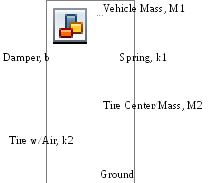A bond graph was created from the model and created in CAMP-G.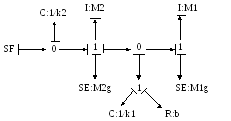Using the MATLAB and SIMULINK programs it was possible extract state space equations and use them in the simulation. Displacement of the tire: dQ2=SF1-P4/I4 Displacement of the vehicle: dQ8=P4/I4-P11/I11 Force on the vehicle: dP11=Q8/C8+P4/I4*R9-P11/I11*R9-SE12 Force on the suspension: dP4=Q2/C2-SE5-Q8/C8-P4/I4*R9+P11/I11*R9 Once the computer model was created and interpreted a simulation could be conducted by setting the initial conditions and entering the design criteria values. This model simulates a vehicle with a mass of 1600kg hitting a bump traveling around 12.5m/s. For this simulation only one wheel was used. The mass of the wheel was set to 12.5kg. The vertical velocity of the ground was used as input for the system. This was calculated by using the trigonometry and the speed of the vehicle. The other equations inherit values from it and the system reacts. The vehicle body is displaced according to the velocity of the body center in relation to the ground. The wheel is displaced according to the velocity of the wheel center also in relation to the ground. The spring constants (k1 and k2) and the damping coefficient (b) were tested with a couple of different values until the suspension looked realistic. Results: Using MATLAB the simulation was conducted with values of the tire spring constant and the suspension spring and damper constants. The following graphs were plotted to get a realistic behavior of the suspension. Using the values: Tire spring constant, k1 = 650kN/m Suspension spring constant, k2 = 250kN/m Suspension damper constant, b = 10,000kg/s This figure shows how the tire and vehicle react to hitting a bump. The first graph shows how the tire compresses, then the suspension takes affect and buffers the impact. The second graph shows how the vehicle body reacts as the suspension compresses at first, then returns to normal. From these graphs, the tire compresses no more than 3cm and the total vertical distance the vehicle is displaced is no more than 10cm.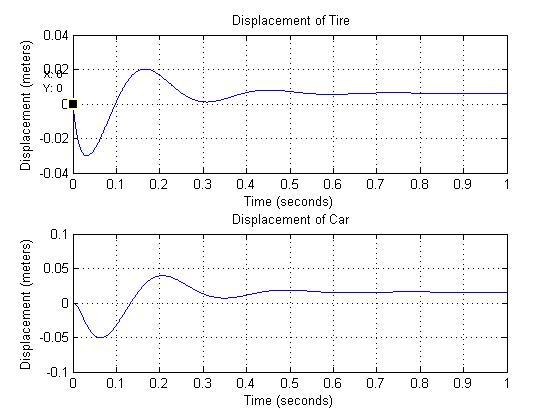The next figure shows the forces acting on the tire and vehicle as it hits a bump.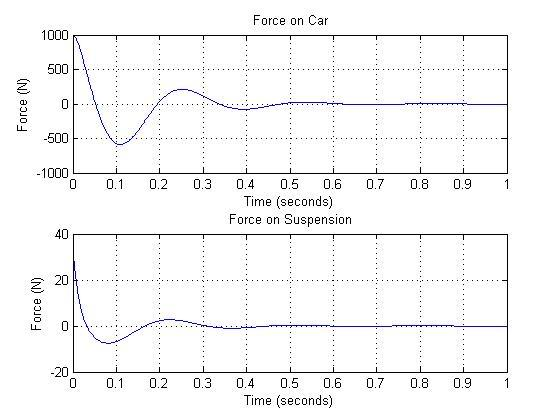Here is the SIMULINK Scope graph of the displacement: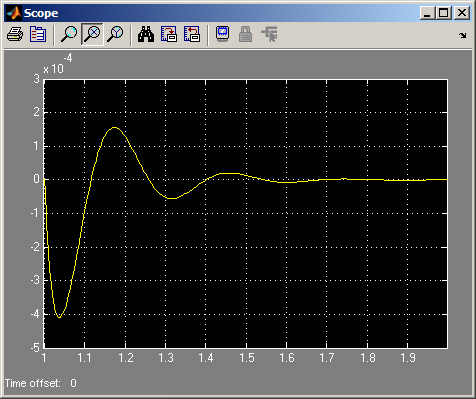Conclusion: The simulation turned out well and was easily used to understand how a vehicle suspension system works. The computer modeling work was easily done with the help of the CAMP-G and MATLAB. They system could have been modeled in more detail, due to time restraints and the amount of lab work the suspension was modeled as most basic system. Future work may include the modeling of a total suspension system including steering, braking, engine torque, body roll, and traction control. Some of the variables were estimated (i.e. vehicle and tire mass and vertical speed). Overall the simulation created realistic values for the behavior of a suspension system. Download 128.09 Kb.Share with your friends: